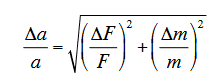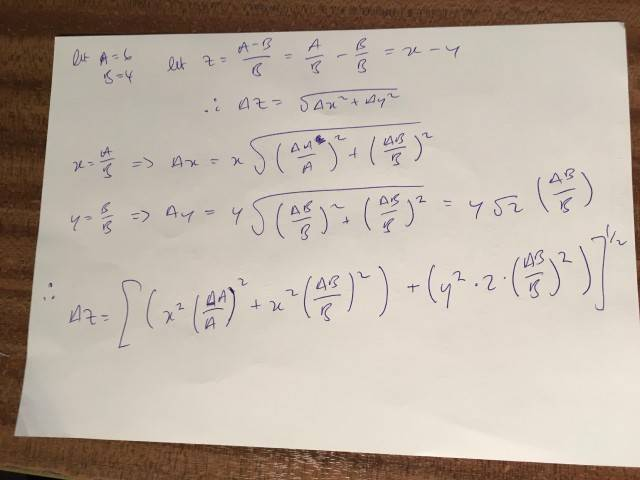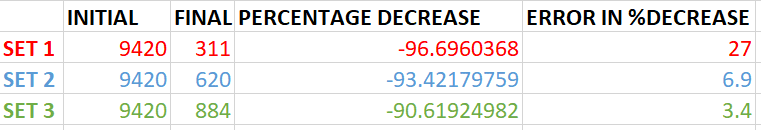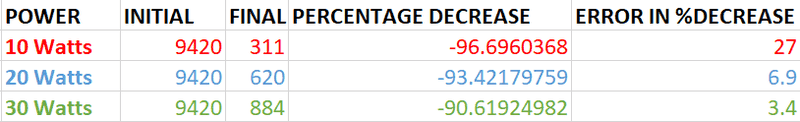# Adding error to a percentage change

• B
This may be a really dumb question and it's been a long day and I can't get my head around this.

If I have two values with errors, say 4 ± 0.5 and 6 ± 1.5, the precentage increase from 4 to 6 is easy its 50 %.

But how do I deal with the errors? I want to write (50 ± X) % where X represents the error in the percentage increase. Do I just take the standard deviation of the two errors so it would be (50.0 ± 0.7) % or do I take an average so its (50 ± 1) %.

Not sure what to do with this, thanks for any pointers.

gleem
But how do I deal with the errors? I want to write (50 ± X) % where X represents the error in the percentage increase. Do I just take the standard deviation of the two errors so it would be (50.0 ± 0.7) % or do I take an average so its (50 ± 1) %.

No. Your starting function is of the form A/B. According to standard procedures you determine the differential of A/B which will be the uncertainty in A/B. Anything look familiar.?

No. Your starting function is of the form A/B. According to standard procedures you determine the differential of A/B which will be the uncertainty in A/B. Anything look familiar.?

Ahhh of course, so something like this...Thanks!

#### Attachments

•gleem
Try again, so like this...Which gives me an error of 0.33, this must be too low? (50.0 ± 0.3) % ??

#### Attachments

Last edited:
gleem
OOPs not quite.get rid of the Δy it is 0.

IF Z = A/B -1

Let ΔA = uncertainty in Z associated with the uncertainty in A = δA The same with ΔB

If δA and δB are random and uncorrelated we may write that ΔZ2 = ΔA2 + ΔB2

What is ΔA? it is (∂Z/∂A)δA The same with ΔB.

You have to be careful in using the correct values for δA and δB .in determining the uncertainty in the percentage change. You must calculate the actual error in Z first then determine the percentage.of ΔZ to Z to get the uncertainty of the percent change of A relative to B.

•rwooduk
mfb
Mentor
No, you have an uncertainty of 0.33 for a value of 0.50. 50%±33%.

Edit: Looks like there is another error somewhere, see the post above. The answer is not too far away from 33%, however.

•rwooduk
Excellent I see now, will have another go tomorrow and shouldn't be a problem, many thanks for the help !

Svein
More than 50 years ago, in my first University physics course, we were taught the basics of measurement errors and how to add them up. In short:
1. Take several measurements
2. The average of the measurements is the best estimate of the "real" value of the thing you are measuring.
3. The standard deviation of the measurements is the best estimate of the absolute measurement error
4. The relative measurement error is the absolute measurement error divided by the average of the measurements
5. If your measurement involves several variables, calculate the average and the relative measurement error for each.
6. You add relative measurement errors in the same way as you add standard deviations - square them, add them and take the square root of the sum.

•rwooduk
This isn't going to work for percentage decrease for fixed error values because if the percentage decrease is greater this will lead to a greater error value!

Example, here is my data:The fixed error for each initial and final value is ± 282.40 therefore for the greatest percentage decrease where it drops to 311 (i.e. for that value it will be 311 ± 282), so the error is huge (± 27) and doesn't reflect the error in the system. The greater the percentage decrease the greater the error will be, so the error now depends on the percentage decrease and not the original standard error. Do you see the problem?

#### Attachments

Svein
The fixed error for each initial and final value is ± 282.40 therefore for the greatest percentage decrease where it drops to 311 (i.e. for that value it will be 311 ± 282), so the error is huge (± 27) and doesn't reflect the error in the system. The greater the percentage decrease the greater the error will be, so the error now depends on the percentage decrease and not the original standard error. Do you see the problem?
Yes, and there is something you do not tell me. Since you have "Initial" and "Final" - is there a time variable involved? What is your measurement - the final value or the decrease? If it is your final values, all you have is an estimate of 605 and an absolute error of 287.

gleem
The fixed error for each initial and final value is ± 282.40 therefore for the greatest percentage decrease where it drops to 311 (i.e. for that value it will be 311 ± 282), so the error is huge (± 27) and doesn't reflect the error in the system. The greater the percentage decrease the greater the error will be, so the error now depends on the percentage decrease and not the original standard error. Do you see the problem?

I do not know what you are doing. You have three measurements. You are looking to determine the percent decrease in the measurements You have two fixed errors for the final and initial measurements. Why didn't you calculate the average decrease and the standard deviation of the data first? You should then incorporate the fixed error to get your total error. Then determine the percentages.

Yes, and there is something you do not tell me. Since you have "Initial" and "Final" - is there a time variable involved? What is your measurement - the final value or the decrease? If it is your final values, all you have is an estimate of 605 and an absolute error of 287.

edit
Apologies, I see where the misunderstanding lies. Each Set is a seperate experiment unrelated to the others. So I need a percentage change and associated error for each set. Edited...So as you can see with a fixed error for each initial and final values (± 282.40), the error becomes dependant upon the amount of decrease (the greater the decrease the more error on the final value) not the standard (± 282.40) error.

For example:

At 10 W the final value is 311 ± 282.40, which will lead to a greater error value for the percentage change (27%) than say for 20 W where the final value is 620 ± 282.40 which gives (6.9%)

The error in the percentage change is dependant upon the amount of change, and does not reflect the systematic ± 282.40 error.

Apologies, hope that makes sense.

#### Attachments

Last edited:
mfb
Mentor
I don't understand how you get your values.
The ratio is final/initial. Its relative uncertainty is sqrt((282.4/final)^2 + (282.4/initial)^2) which is about 90%, 46% and 32%, respectively. The absolute uncertainty for the ratio is then that number multiplied by the ratio - and it is about 0.03 in all cases. The absolute uncertainty for 1-ratio is the same. You get 3% uncertainty for the percent decrease.

•rwooduk
I don't understand how you get your values.
The ratio is final/initial. Its relative uncertainty is sqrt((282.4/final)^2 + (282.4/initial)^2) which is about 90%, 46% and 32%, respectively. The absolute uncertainty for the ratio is then that number multiplied by the ratio - and it is about 0.03 in all cases. The absolute uncertainty for 1-ratio is the same. You get 3% uncertainty for the percent decrease.

Sorry, yes I had derived the uncertainty formula, then things have gone wrong somewhere, you are right, but I will recheck my calculations.

Thanks again for the help ! Three years of chemistry and I've completely forgotten my physics !! Thanks again